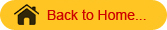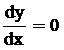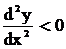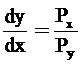## MATHS :: Lecture 17 :: Physical and Ecomonic OptimumPhysical and Economic Optimum for single input
Let y = f(x) be a response function. Here x stands for the input that is kgs of fertilizer applied per hectare and y the corresponding output that is kgs of yield per hectare.
We know that the maximum is only whenand.
This optimum is called physical optimum. We are not considering the profit with respect to the investment, we are interested only in maximizing the profit.
Economic optimum
The optimum which takes into consideration the amount invested and returns is called the economic optimum.where Px → stands for the per unit price of input that is price of fertilizer per kgs.
Py → stands for the per unit price of output that is price of yield per kgs.